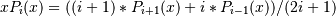# numpy.polynomial.legendre.legmulx¶

numpy.polynomial.legendre.legmulx(c)[source]

Multiply a Legendre series by x.

Multiply the Legendre series c by x, where x is the independent variable.

Parameters: c : array_like 1-D array of Legendre series coefficients ordered from low to high. out : ndarray Array representing the result of the multiplication.

Notes

The multiplication uses the recursion relationship for Legendre polynomials in the form#### Previous topic

numpy.polynomial.legendre.legmul

#### Next topic

numpy.polynomial.legendre.legdiv